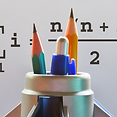# Math Unmuddled: IntegersThe ‘Math Unmuddled’ series takes up topics that pose common difficulties for children and clarifies these to build strong conceptual foundations.

A good grasp of basic Mathematics is important in today’s world, irrespective of the career one chooses to pursue. While Math is typically associated with Science and Engineering, it is hard to think of any line of work that does not involve an understanding of school-level Math (at least till grade 10). Unfortunately, many students struggle with the subject and even develop a fear of Math. The most common reason for this is that they attempt to remember many disconnected rules and procedures, and this makes Math seem more difficult than it is.

The GenWise team has seen many instances of this in their experience. For example, we have asked grade 2/3 students to perform a division like 391/17. After they answer this correctly and say ‘23”, we ask them to multiply 23 by 17. We find that there are always some students who start performing this multiplication instead of immediately seeing that the answer has to be 391 (because multiplication is the inverse of division). When underlying concepts such as these are understood, there is ‘less to remember’ and Math becomes simple and unmuddled. The ‘Math Unmuddled’ series takes up topics that pose common difficulties for children and clarifies these to build strong conceptual foundations.

This course in the series focuses on clarifying integers and integer operations. Misconceptions can form easily when children are introduced to integers, because children must be able to refine and redefine concepts they have learned in earlier classes. For example, they might have learned in lower classes that the smaller number has to be subtracted from the larger number. But once negative numbers are introduced, the above rule becomes redundant.

If your child is not able to perform integer operations quickly and correctly or is unable to explain why the rules he/she is following make sense, then the course will be helpful. Shared below are some questions which sometimes confuse young students.

• (-2) - (-4) =  ____  = (-4) - ____
• 12 - 27 = ____ = ___ - 27
• Give an example of a real life situation that represents 4-10 = -6
• Place these numbers in order of largest to smallest: .00156, 1/60, .0015, .001, .002

The course is based on what research says about common errors and difficulties students face in working with integers and covers the following areas-

• Why negative numbers and where they appear in real life
• Integers on a number line
• Visualisation to understand the four operations
• The subtraction operation and the negative number
• Translation of English to Math

Note:

The course is not a substitute for learning the topic in school. It is assumed that students already have some familiarity with the topic. Also, in the limited time available in this course, it is not possible to focus on practice. Students must internalize what they learn in the course through subsequent practice, under the guidance of a teacher or a parent.

References: